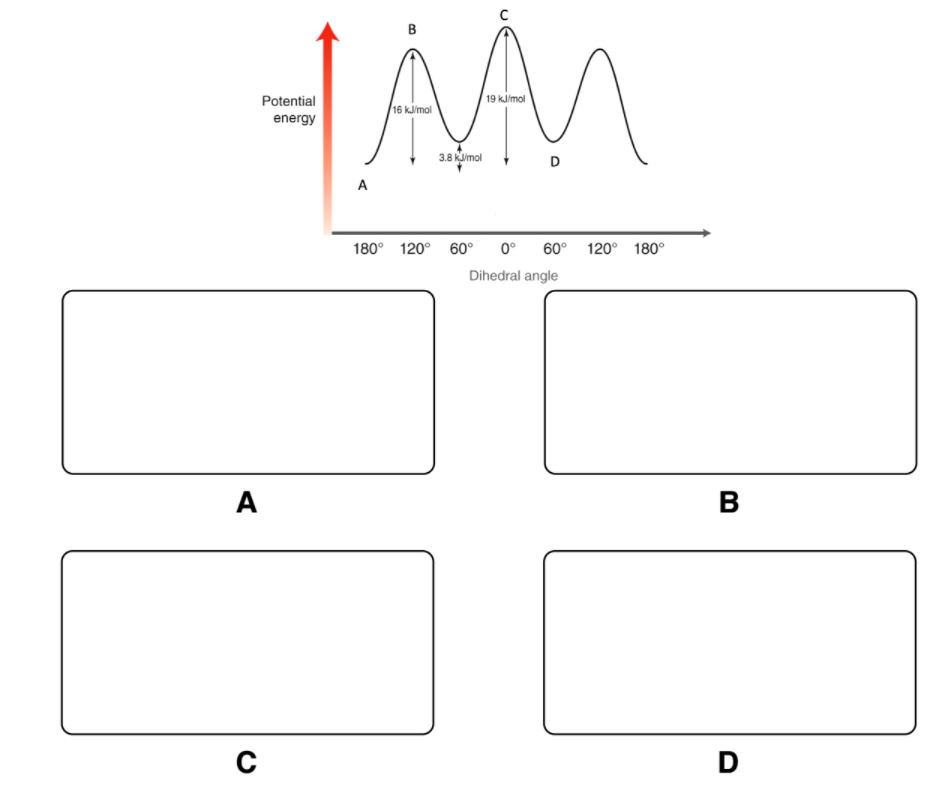# Problem: Conformational analysis is the study of the energy changes (stability) of different spatial arrangements of atoms relative to rotations about single bonds. Perform the following analyses:Using Newman Projections, fill in the potential energy diagram for the rotation around the C2-C3 bond in butane (i.e. draw each Newman Projection onto the diagram at each angle of rotation specified). ]

###### Problem Details

Conformational analysis is the study of the energy changes (stability) of different spatial arrangements of atoms relative to rotations about single bonds. Perform the following analyses:

Using Newman Projections, fill in the potential energy diagram for the rotation around the C2-C3 bond in butane (i.e. draw each Newman Projection onto the diagram at each angle of rotation specified). ]